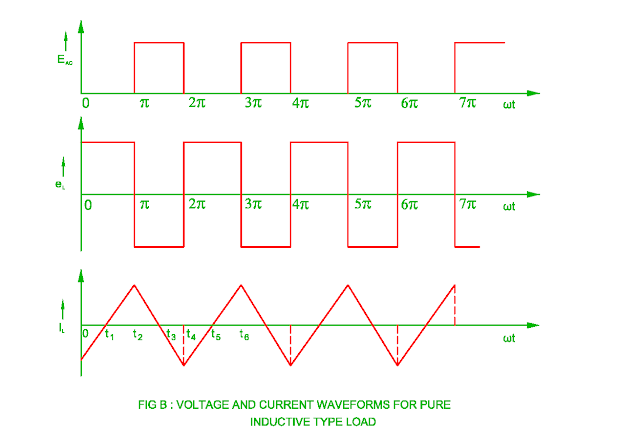## 22/05/2017

### Voltage Source Inverter ( VSI )

• The basic circuit for voltage driven inverter is shown in the figure A. The DC supply is given to the primary winding of the transformer.
• There are two semiconductor switches S1 and S2 in which switching is done by astable multivibrator at selected frequency.

• When the switch S1 is kept on, the current flows through transformer upper primary winding resulting the flux generates in the core and induced emf in it.
• Similarly when switch S2 is kept on, the current flows through lower part of the transformer primary winding resulting the flux generates in the reverse direction.
• This will result in emf induced in the reverse direction. The switching of switch S1 and S2 are done alternately.
• There is a transistor, SCR, MOSFET, IGBT or GTO used as a semiconductor device.
• The saturation voltage drop of these devices is low therefore it is neglected. As the input voltage is DC, the output is square wave.
Effect of Type of Load on Output of Inverter

• The waveform of the voltage for the voltage source inverter is square whereas the waveform of the current depends upon type of load.

• When the load is pure resistive type, the voltage and current waveform are in the phase therefore the current waveform is also square waveform.
• The conduction of switch S1 and S2 is done for half cycle or 1800.

• When the load is inductive type in the voltage source inverter, the voltage waveform always square wave, but current waveform lags 900 with respect to voltage waveform.
• The power flows from supply to load side during t1 to t2 time whereas it flows from load to supply side from time t2 to t3
• It means that when the load voltage and load current both are either positive or negative, the power flows from supply to load side whereas either the load voltage or load current negative resulting power flows from load to supply side.
• When a semiconductor switch S1 is turned off, the direction of current does not change at instant.
• When the load current becomes maximum, it is interrupted resulting a switching semiconductor device may damaged.
• There is same problem arise when a switching semiconductor device S2 is turned off at π time.This problem is solved by

•  A resistance is connected across the load. This resistance dissipates energy of inductor. This is called as Preloading of resistor
• The size of inverter increases due to resistor and inverter efficiency decreases.

(2) The RC circuit is connected across the load resulting the efficiency of the inverter decreases.
(3) The reverse voltage spike is reduced by back to back zener diode during switching operation.

(4) One method of reducing voltage spike is shown in the figure D. The primary of transformer consists of two center taped winding. There are two diodes connected in the one center taped winding. When a semiconductor switch S1 and S2 are kept off, conduction of diode starts and energy transfers towards supply side.